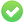# high precision, calculate correction value

hi @all,

a question: ‘5 minutes’ represented as a fraction of a day (represented by ‘1’) are an ‘odd’ ‘uneven’ - ‘endless fraction’ value, already in decimals, and also in doubles (can be calculated as '=time(0;5;0),

thus there is a gap between the value calc can deal with (’=1/24/60*5’ or ‘=1/24/12’, or ‘=1/288’, all resulting in ‘0,00347222222222222’) and the ideal precise value of 0,003472222222222222222222222222222… , something like 0,000000000000000002222222222222222… or - as calc has one digit more hidden from users view: 0,000000000000000000222222222222222…

i can! calculate that gap quite precise to 1.9274705288631189921192130137104208E-19 with the 128 bit variant of ‘weitz’ (www.weitz.de/ieee), doe’s anybody have an idea how i can get that value in a calc sheet? wouldn’t need 35 digit precision, 15 or 16 digits acc. normal double is sufficient, just 10 digit could be enough.

i know the value cannot be added to the 5 minute value as it’s beyond it’s ULP, but if one can get such correction values i see some nice possibilities to do correct calculations.

let’s say: 1/288 is a fraction which we know, exact, we can check that calc calculates with something like '0,0034722222222222220294, how to calculate the difference ‘in the sheet’?

(sorry, i am! somewhat overworked, normally i should know such things by myself … let me change the question … what is the best - most elegant - way do get such values ?)

Numbers in most spreadsheets are limited to 15 significant digits. If you need better precision, don’t think about spreadsheet, that’s all.

To show the community your question has been answered, please tag the best answer (), and/or vote for any helpful answer. Else, please edit your question to add information (answers are for solutions only).

@Hagar_Delest: this limit in precision often accumulates and then affects digits further ahead, which is annoying for some tasks. Against this i need the correction value. Since calc can! store the correction value (calc also can deal with much smaller numbers) and also can work with it i think there might be a trick to get it calculated …

My point is that spreadsheets are not the best tools if you need such precise calculations. Even if you store a correction value, using them may trigger similar calculation limitations.

@Hagar_Delest: i know… and that’s what i’m researching for: which errors are unavoidable, and which can be circumvented,
in nowadays life there is hardly another choice than spreadsheets for plenty tasks, and i’d like them to calculate as precise as possible … for that: do you have any idea to calculate that small deviation?

No idea.

You can ask the question in the forum also (see link in my profile), there are Calc gurus also.